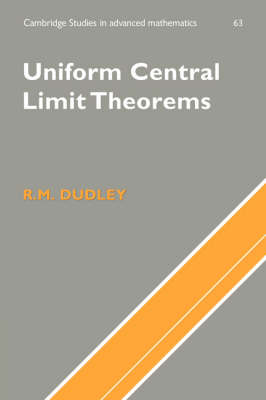•# Uniform Central Limit Theorems - Cambridge Studies in Advanced Mathematics No.63 (Hardback)

(author)
£94.00
Hardback Published: 28/07/1999
• Not available

This product is currently unavailable

This product is currently unavailable.

This book shows how the central limit theorem for independent, identically distributed random variables with values in general, multidimensional spaces, holds uniformly over some large classes of functions. The author, an acknowledged expert, gives a thorough treatment of the subject, including several topics not found in any previous book, such as the Fernique-Talagrand majorizing measure theorem for Gaussian processes, an extended treatment of Vapnik-Chervonenkis combinatorics, the Ossiander L2 bracketing central limit theorem, the Gine-Zinn bootstrap central limit theorem in probability, the Bronstein theorem on approximation of convex sets, and the Shor theorem on rates of convergence over lower layers. Other results of Talagrand and others are surveyed without proofs in separate sections. Problems are included at the end of each chapter so the book can be used as an advanced text. The book will interest mathematicians working in probability, mathematical statisticians and computer scientists working in computer learning theory.

Publisher: Cambridge University Press
ISBN: 9780521461023
Weight: 730 g
Dimensions: 228 x 152 x 30 mm